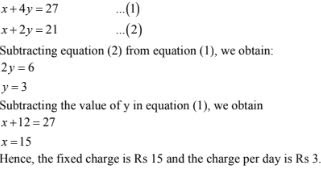# RD Sharma Solutions Chapter 3 Pair of Linear Equation in Two Variables Exercise 3.6 Class 10 Maths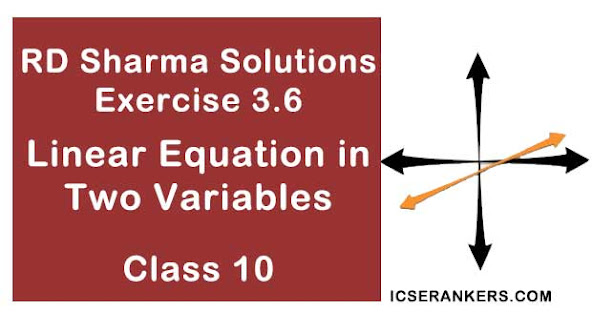Chapter Name RD Sharma Chapter 3 Pair of Linear Equation in Two Variables Book Name RD Sharma Mathematics for Class 10 Other Exercises Exercise 3.1Exercise 3.2Exercise 3.3Exercise 3.4Exercise 3.5 Related Study NCERT Solutions for Class 10 Maths

### Exercise 3.6 Solutions

1. 5 pens and 6 pencils together cost Rs 9 and 3 pens and 2 pencils cost Rs 5. Find the cost of 1 pen and 1 pencil.
Solution

Let the cost of a pen be Rs x and that of a pencil be Rs y. Then,
5x + 6y = 9 ...(i)
and  3x + 2y = 5 ...(ii)
Multiplying equation (i) by 2 and equation (ii) by 6, we get
10x + 12y = 18 ...(iii)
18x + 12y = 30 ...(iv)
Subtracting equation (iii) by equation (iv), we get
18x - 10x + 12y - 12y = 30 - 18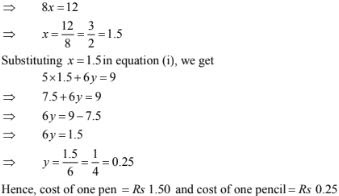2. 7 audio cassettes and 3 video cassettes cost Rs 1110, while 5 audio cassettes and 4 video cassettes cost Rs 1350. Find the cost of an audio cassette and a video cassette.
Solution

Let the cost of a audio cassette be Rs x and that of a video casette be Rs y. Then,
7x + 3y = 1110 ...(i)
and 5x + 4y = 1350 ...(ii)
Multiplying equation (i)  by 4 and equation (ii) by 3, we get
28x + 12y = 4440 ...(iii)
15x + 12y = 4050 ...(iv)
Subtracting equation (iv) from equation (iii), we get
28x - 15x + 12y - 12y = 4440 - 4050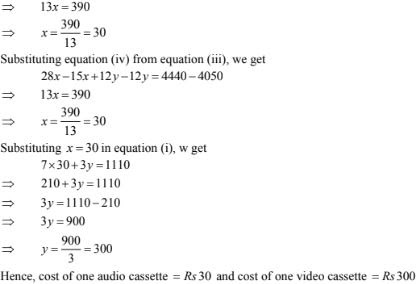3. Reena has pens and pencils which together are 40 in number. If she has 5 more pencils and 5 less pens, then number of pencils would become 4 times the number of pens. Find the original number of pens and pencils.
Solution
Let the number of pens be x and that of pencil be y, then,
x + y = 40 ...(i)
and (y +5) = 4(x - 5)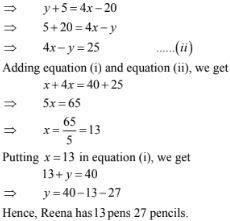4. 4 tables and 3 chairs, together, cost Rs 2,250 and 3 tables and 4 chairs cost Rs 1950. Find the cost of 2 chairs and 1 table.
Solution

Let the cost of a table be Rs x and that of a chairs be Rs y. Then,
4x + 3y = 2,250 ...(i)
and, 3x + 4y = 1950 ...(ii)
Multiplying equation (i) by 4 and equation (ii) by 3, we get
16x + 12y = 9000 ...(iii)
9x + 12y = 5850 ...(iv)
Subtracting equation (iv) by equation (iii), we get
16x - 9x  = 9000 - 5850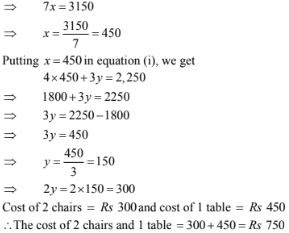5. 3 bags and 4 pens together cost Rs 257  whereas 4 bags and 3 pens together cost Rs 324. Find the total cost of 1 bag and 10 pens.
Solution

Let the cost of a bag be Rs x and that of a pen be Rs y. Then,
3x + 4y = 257 ...(i)
and, 4x + 3y = 324  ...(ii)
Multiplying equation (i) by 3 and equation (ii) by 4, we get
9x + 12y = 770 ...(iii)
16x + 12y = 1296 ...(iv)
Subtracting equation (iii) by equation (iv), we get
16x - 9x = 1296 - 771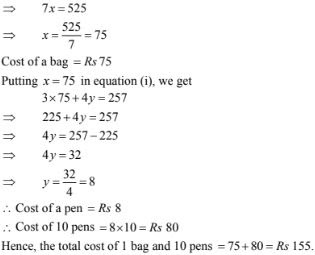6. 5 books and  7 pens together cost Rs79 whereas 7 books and 5 pens together cost Rs 77. Find the total cost of 1 book and 2 pens.
Solution

Let the cost of a book be Rs x and that of a pen be Rs y. Then,
5x + 7y = 79 ...(i)
and 7x + 5y = 77  ...(ii)
Multiplying equation (i) by 5 and equation (ii) by 7, we get
25x + 35y = 395 ...(iii)
49x + 35y = 539  ...(iv)
Subtracting equation (iii) by equation (iv), we get
49x - 25x = 539 - 395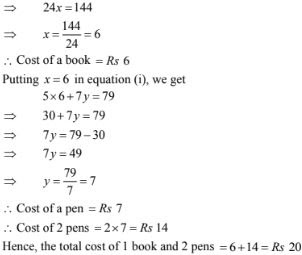7. A and B each have a certain number of mangoes. A says to B, " if you give 30 of your mangoes. I will have twice as many as left with you." B replies, " if you give me 10, I will have thrice as many as left with you." How many mangoes does each have?
Solution

Suppose A has x mangoes and B has y mangoes
According to the given conditions, we have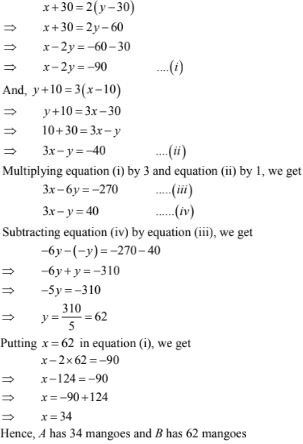8. On selling a T.V. at 5% gain and a fridge at 10% gain, a shopkeeper gains Rs 2000. But if he sells the T.V. at 10% gain and the fridge at 5% loss. He gains Rs 1500 on the transaction. Find the actual prices of T.V.  and fridge.
Solution

Let the price of a T.V. be Rs x and that of a fridge be Rs y. Then, we have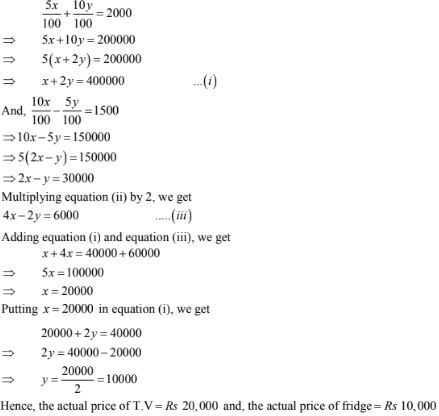9. The coach of a cricket team buys 7 bats and 6 balls for Rs 3800. Later, he buys 3 bats and 5 balls for Rs 1750. Find the cost of each bat and each ball .
Solution
Let the cost of bat and a ball be x and y respectively
According to the given information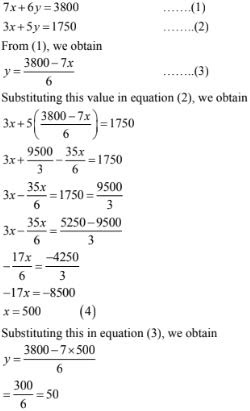Hence, the cost of a bat is Rs 500 and that of a ball is Rs 50.
Concept Insight: Cost of bats and balls needs to be found so the cost of a ball and bat will be taken as the variables. Applying the conditions of total cost of bats and balls algebraic equations will be obtained. The pair of equations can  then be solved by suitable substitution.

10. One says, " Give me a hundred, friend ! I shall then become twice as rich as you." The other replies, " If you give me ten, I shall be six times as rich as you. " Tell me what is the amount of their respective capital?
Solution

Let the money with the first person and second person be Rs x and Rs y respectively.
According to the question

>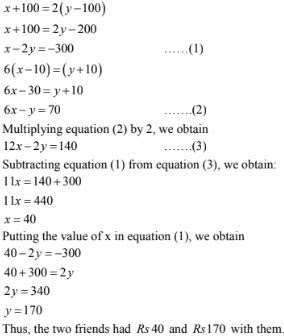11. A lending library has a fixed charge for the first three days and an additional charge for each day thereafter. Saritha paid Rs 27 for a book kept for seven days, while Susy paid Rs 21 for the book she kept for five days. Find the fixed charge and the charge for each extra day.
Solution

Le the fixed charge for first three days and each day charge thereafter be Rs x and Rs y  respectively.
According to the question,